How Cheenta works to ensure student success?
Explore the Back-Story

# TIFR 2014 Problem 21 Solution - Determining functionTIFR 2014 Problem 21 Solution is a part of TIFR entrance preparation series. The Tata Institute of Fundamental Research is India's premier institution for advanced research in Mathematics. The Institute runs a graduate programme leading to the award of Ph.D., Integrated M.Sc.-Ph.D. as well as M.Sc. degree in certain subjects.
The image is a front cover of a book named Introduction to Real Analysis by R.G. Bartle, D.R. Sherbert. This book is very useful for the preparation of TIFR Entrance.

Also Visit: College Mathematics Program of Cheenta

## Problem:

Let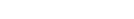be continuous. Suppose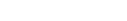for all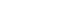.

Then

A. no such function exists

B. there are infinitely many such functions

C. there is only one such function

D. there are exactly two such functions

Discussion:

Basically, the question is to find out how many such functions can exist.

Let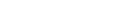. That is,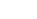is the indefinite integral of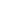.

We know from the fundamental theorem of calculus that: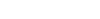.

So we have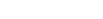for all.

We will manage this equation as we do in case of differential equations. Except that, we have an inequality here.for all.

Multiplying both sides by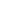the inequality remain unchanged. This is because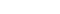.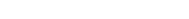for all.

Now,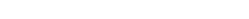.

So we have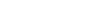for all.

Therefore, by taking integral from o to y and by using fundamental theorem of calculus: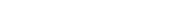for all.

i.e,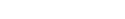for all.

Also, note that by definition of (F), (F(0)=0). So we have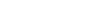for all.

But, sincefor all, we have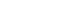for all.

So far, we have not used the fact thatis a non-negative function. Now we use it. Since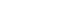for all, therefore by monotonicity of the integral,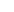is an increasing function. This meansfor all.

By the two inequalities obtained above, we getfor all.

By the fundamental theorem (again!) we get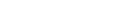for all.

So there is only one suchnamely the constant function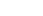.

## Helpdesk

• What is this topic: Real Analysis
• What are some of the associated concept: Fundamental theorem of calculus, Increasing Function
• Book Suggestions: Introduction to Real Analysis by R.G. Bartle, D.R. SherbertTIFR 2014 Problem 21 Solution is a part of TIFR entrance preparation series. The Tata Institute of Fundamental Research is India's premier institution for advanced research in Mathematics. The Institute runs a graduate programme leading to the award of Ph.D., Integrated M.Sc.-Ph.D. as well as M.Sc. degree in certain subjects.
The image is a front cover of a book named Introduction to Real Analysis by R.G. Bartle, D.R. Sherbert. This book is very useful for the preparation of TIFR Entrance.

Also Visit: College Mathematics Program of Cheenta

## Problem:

Letbe continuous. Supposefor all.

Then

A. no such function exists

B. there are infinitely many such functions

C. there is only one such function

D. there are exactly two such functions

Discussion:

Basically, the question is to find out how many such functions can exist.

Let. That is,is the indefinite integral of.

We know from the fundamental theorem of calculus that:.

So we havefor all.

We will manage this equation as we do in case of differential equations. Except that, we have an inequality here.for all.

Multiplying both sides bythe inequality remain unchanged. This is because.for all.

Now,.

So we havefor all.

Therefore, by taking integral from o to y and by using fundamental theorem of calculus:for all.

i.e,for all.

Also, note that by definition of (F), (F(0)=0). So we havefor all.

But, sincefor all, we havefor all.

So far, we have not used the fact thatis a non-negative function. Now we use it. Sincefor all, therefore by monotonicity of the integral,is an increasing function. This meansfor all.

By the two inequalities obtained above, we getfor all.

By the fundamental theorem (again!) we getfor all.

So there is only one suchnamely the constant function.

## Helpdesk

• What is this topic: Real Analysis
• What are some of the associated concept: Fundamental theorem of calculus, Increasing Function
• Book Suggestions: Introduction to Real Analysis by R.G. Bartle, D.R. Sherbert

This site uses Akismet to reduce spam. Learn how your comment data is processed.

### One comment on “TIFR 2014 Problem 21 Solution - Determining function”

1.rajarshi says:

can we apply liebnitz rule at the first step ?

### Knowledge Partner